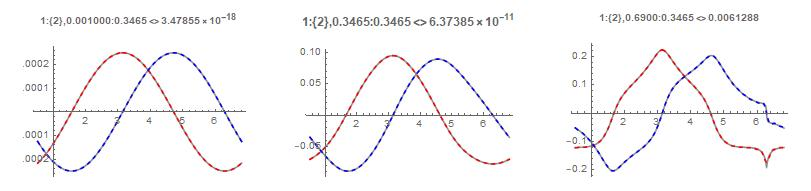### Section 14: Analyzing the Annular Puiseux Power Series

$$\newcommand{\bint}{\displaystyle{\int\hspace{-10.4pt}\Large\mathit{8}}} \newcommand{\res}{\displaystyle{\text{Res}}}$$

1. Background
2. The reader is asked to review Sections 10 and 13 for background on this section.

In this section we study the power expansions of annular algebraic branches. In Section 13, we derived the integral expressions for computing the Puiseux expansions of an algebraic branch. We briefly summarize that derivation here. Given an $n$-cycle branch, $w_n(z)$, the power expansion is given by: \begin{equation} \begin{aligned} w_n(z)&= \sum_{k=0}^{\infty} a_k (z^{1/n})^k+\sum_{k=1}^{\infty} \frac{b_k}{\left(z^{1/n}\right)^k} \\ &=A(z)+S(z) \end{aligned} \label{equation:equation001} \end{equation} with \begin{equation} \begin{aligned} a_k&=\frac{1}{2n\pi i} \bint \frac{w_n(z)}{\left(z^{1/n}\right)^{k+n}} dz\\ b_k&=\frac{1}{2n\pi i} \bint w_n(z)\left(z^{1/n}\right)^{k-n} dz.\\ \label{equation:equation4} \end{aligned} \end{equation} and $A(z)$ being the analytic terms of the series and $S(z)$, the singular terms. Or in symmetrical form: \begin{equation} \begin{aligned} a_k&=\frac{1}{2n\pi i} \bint \frac{w_n(z)}{\left(z^{1/n}\right)^{k+n}} dz\\ w_n(z)&=\sum_{p=-\infty}^\infty a_p (z^{1/n})^p. \end{aligned} \label{equation:equation100} \end{equation}

We again use the function studied in sections 10 and 13: \begin{equation} \begin{aligned} f(z,w)&=(-z^2+z^3)\\ &+(-4 z+3 z^2)w\\ &+(-z^3-9 z^4)w^2\\ &+(-2+8 z+4 z^2-4 z^3)w^3\\ &+(6-8 z^2+7 z^3+8 z^4)w^4 \end{aligned} \label{equation:equation20} \end{equation} In Section 10, we computed the branch continuation table for this function. In Section 13, we analyze the integral expression of (\ref{equation:equation100}) in the following form: \begin{equation} \begin{aligned} c_k&=\frac{1}{2n\pi i} \bint \frac{w_n(t)}{\left(z^{1/n}\right)^{k+n}} dz=\frac{1}{2 n \pi} \int_{t_0}^{t_e} \frac{w_n(t)re^{it}}{\left(re^{it}\right)^{\frac{k+n}{n}}}\\ &=\frac{1}{2 n\pi} \int_{t_0}^{t_e} w_n(t) \left(re^{it}\right)^{-k/n} dt\\ &=\frac{1}{2 n\pi r^{k/n}} \int_{t_0}^{t_e} w_n(t)\big[\cos(tk/n)-i\sin(tk/n)\big] dt\\ &=\frac{1}{2 n\pi r^{k/n}} I(k,n), \end{aligned} \label{eqn5} \end{equation} where as we've discussed, the path of integration from $t_0$ to $t_e$ is along a closed, analytically-continuous $2n\pi$ loop around the branch surface of $w_n$ usually with $r$ in the middle of the branch annular domain.

3. Computing the integral Puiseux expressions
4. Consider the first ring of branches. From Section $13$, we determined the first ring has one $2$-cycle and two single cycle branches with the $\{2\}$ branch extending to the fourth ring with radius $0.69$. We compute the power series for the $\{2\}$ branch using Newton Polygon and using Equation $\ref{eqn5}$ with integration parameters StepSize=1/1000 and Precision=30 with $r$ set to $\frac{173229}{500000}$ being a rational approximation to the center of the annular region of this branch (recall we use rational expressions for calculations to maintain arbitrary precision calculations where possible). The results are given in Table 1 (the values are truncated for brevity) The primary purpose of Table (1) is to first confirm our calculations are correct (compared to the Newton Polygon results), and secondly, to show how the numerical precision decreases as we integrate increasingly oscillatory integrands (See Section 13 for more information about this). This decreasing precision is reflected in the last column which shows the difference between the two calculations increasing from $10^{-16}$ to $10^{-7}$. Of course we could improve this by increasing the precision and decreasing the step size of the computation with a corresponding larger run time as was shown in Section 13.

 Exponent Newton Polygon Integral Series Difference $1$ $-0.25$ $-0.25 - 8.84*10^{-18}i$ $3.33*10^{-16}$ $2$ $0.0703125$ $0.0703125 + 1.14*10^{-18}i$ $1.58*10^{-15}$ $3$ $0.02075$ $0.02075 + 3.22*10^{-17}i$ $2.20*10^{-15}$ $4$ $0.003997$ $0.003997 - 2.88*10^{-17}i$ $1.6291*10^{-15}$ $5$ $-0.106094$ $-0.106094 + 1.165*10^{-17}i$ $9.2481*10^{-14}$ $6$ $0.01224$ $0.01224 + 3.517*10^{-16}i$ $2.325*10^{-14}$ $7$ $-0.0184$ $-0.0184 - 1.260*10^{-15}i$ $6.2199*10^{-14}$ $8$ $-0.0385$ $-0.0385 - 2.369*10^{-14}i$ $2.422*10^{-13}$ $9$ $-0.1234$ $-0.1234- 5.9428*10^{-15}i$ $1.0893*10^{-12}$ $10$ $-0.03308$ $-0.03308 + 4.680*10^{-14}i$ $4.473*10^{-13}$ $11$ $-0.0934$ $-0.0934 - 5.0926*10^{-14}i$ $2.2042*10^{-12}$ $12$ $-0.1397$ $-0.1397 - 1.0623*10^{-12}i$ $4.548*10^{-12}$ $13$ $-0.2387$ $-0.2387 + 1.6253*10^{-12}i$ $9.967*10^{-12}$ $14$ $-0.17635$ $-0.17635 + 1.8414*10^{-12}i$ $4.85*10^{-12}$ $15$ $-0.3186$ $-0.3186 + 2.559*10^{-12}i$ $2.744*10^{-11}$ $16$ $-0.4422$ $-0.4422 + 7.5422*10^{-11}i$ $1.0890*10^{-10}$ $17$ $-0.6288$ $-0.6288 - 2.413*10^{-11}i$ $1.124*10^{-10}$ $18$ $-0.6792$ $-0.6792 + 7.2183*10^{-11}i$ $1.4129*10^{-10}$ $19$ $-1.05701$ $-1.05701 - 7.136*10^{-10}i$ $8.33786*10^{-10}$ $20$ $-1.43468$ $-1.43468 + 4.04889*10^{-9}i$ $4.050*10^{-9}$ $21$ $-1.9564$ $-1.9564 - 2.653*10^{-10}i$ $9.7433*10^{-9}$ $22$ $-2.4597$ $-2.4597+ 3.5199*10^{-9}i$ $2.4333*10^{-8}$ $23$ $-3.58262$ $-3.58262 + 8.3215*10^{-9}i$ $1.2434*10^{-8}$ $24$ $-4.858$ $-4.858 - 3.1973*10^{-7}i$ $5.435*10^{-7}$ $25$ $-6.6196$ $-6.6196 - 4.914*10^{-7}i$ $5.63925*10^{-7}$

Continuing with the $\{2\}$ branch in annulus one, we now wish to study how accurate the power series generated by Equation $\ref{eqn5}$ represents the function along this and other branches.

1. Ring $1$ branch $\{2\}$ series
2. This branch has a domain range of approx. $(0,0.693)$. We generate the first 51 terms of the power series for the branch. We then compute the value of the branch near the lower domain limit, in the middle of the domain, and near the upper domain limit using $z(t)=r_i e^{it}$ with $r_{1}=0.01, r_2=0.35, r_3=0.68$ using two methods: the trace function used to create the power series, and the actual power series. We then compute the error integral between the two: $$e=\int_{t_0}^{t_e}\big|\text{series}(z)-\text{trace}(z)\big|dt$$ The results of these calculations are shown in the figure below. The title for the first plot (and others) is $1:2,0.001:0.3465<>3.48\times 10^{-18}$. This represents ring $1$ branch $2$ with series trace of 0.3465 (center of domain) and branch trace of $0.001$ using the calculated series. The difference between the actual value of the branch along the path $z(t)=0.001 e^{it}$ and the value computed by the power series was on the order of $e=10^{-18}$. In the plot we show the real value of the trace results as the underlying grey plot and the real part of the series results superimposed over the grey plot as the red dashed plot. The blue plot is the imaginary results. These plots show the series computed by the integral method above agrees well with the actual (numerical) value of the branch.3. Ring $1$ branch $\{1,3\}$ series
4. For this branch, we compute $20$ terms of the series and generate the results below. One may be tempted to believe there is a discontinuity in the third plot arount $t=9$ where there appears to be a sharp peak. This is not really a peak but rather the trace coming close to the singular point at $z=-0.009$ where the function values rises sharply along the surface of the pole, reaches the maximum value, then drops quickly.